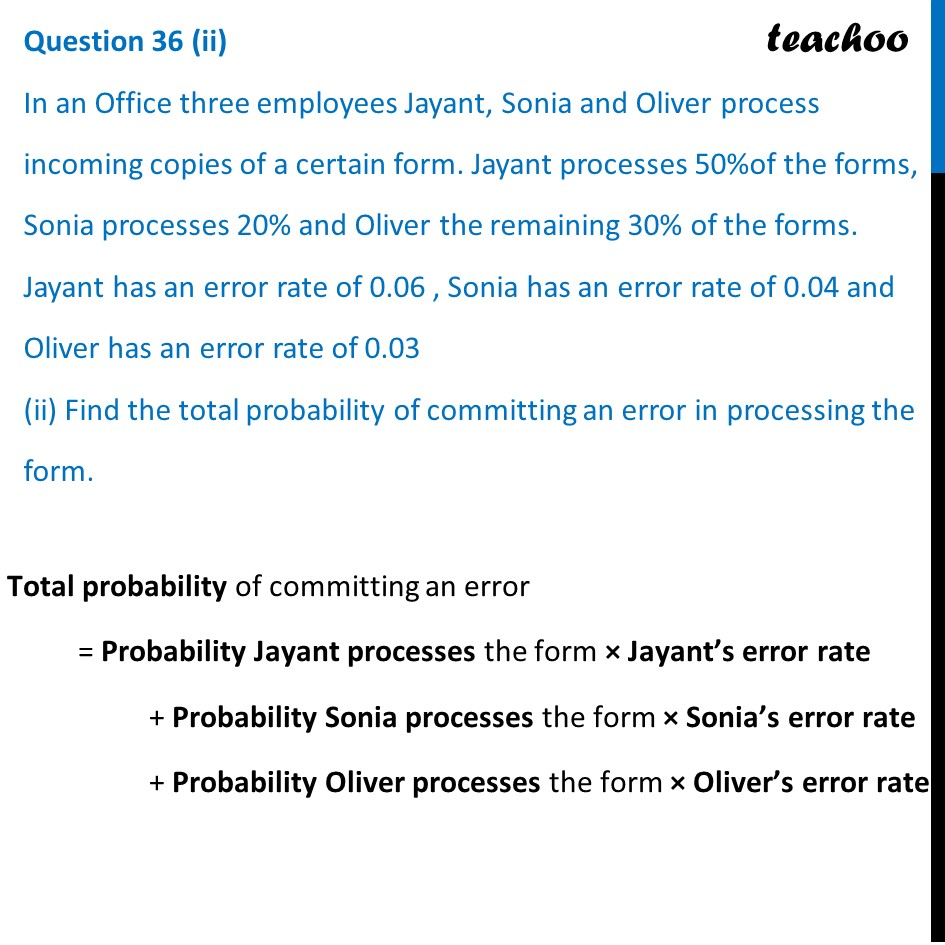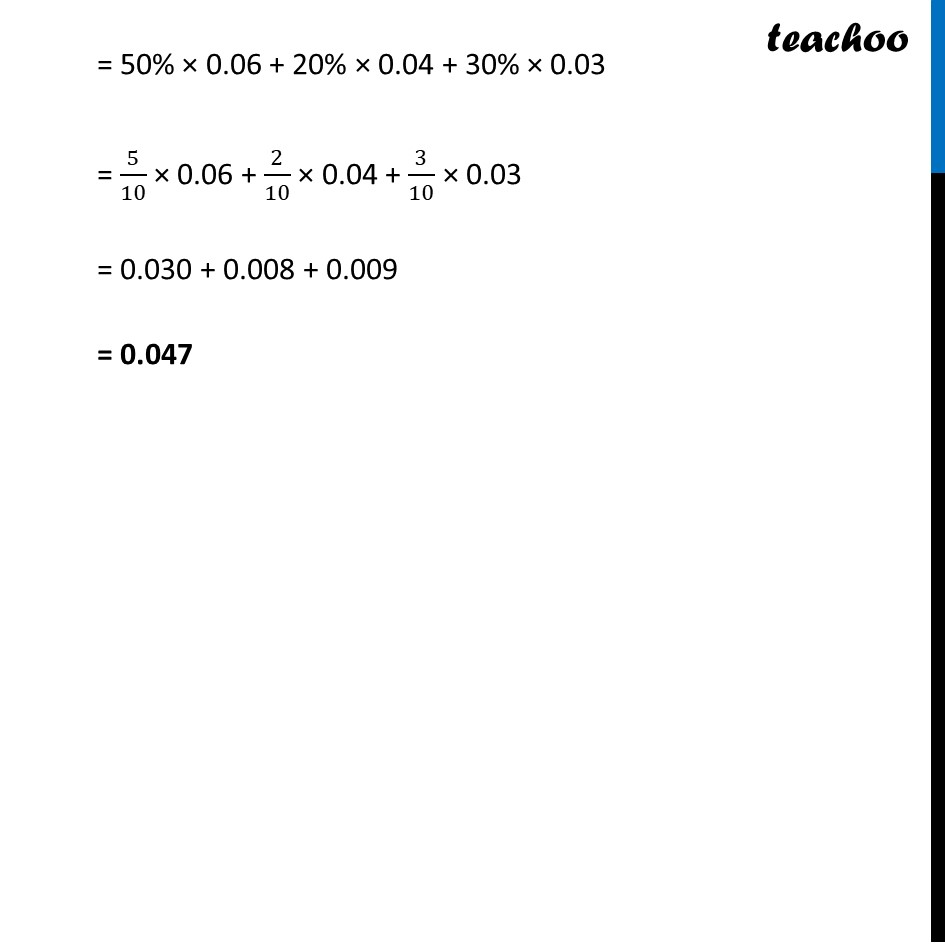CBSE Class 12 Sample Paper for 2024 Boards

Class 12
Solutions of Sample Papers and Past Year Papers - for Class 12 Boards

## (ii) Find the total probability of committing an error in processing the form.Learn in your speed, with individual attention - Teachoo Maths 1-on-1 Class

### Transcript

Total probability of committing an error = Probability Jayant processes the form × Jayant’s error rate + Probability Sonia processes the form × Sonia’s error rate + Probability Oliver processes the form × Oliver’s error rate = 50% × 0.06 + 20% × 0.04 + 30% × 0.03 = 5/10 × 0.06 + 2/10 × 0.04 + 3/10 × 0.03 = 0.030 + 0.008 + 0.009 = 0.047